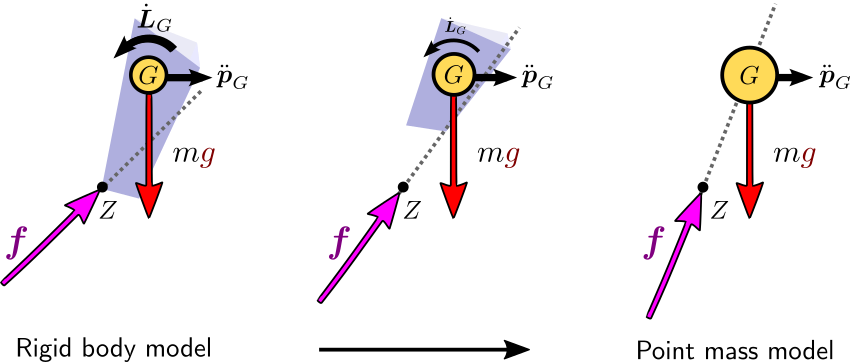# Point mass model

The point mass model is a recurring reduced model in physics in general, and in locomotion modeling in particular. It is a common ancestor to the inverted pendulum and linear inverted pendulum models. In this post, we will review the assumptions that define it, and some of their not-so-intuitive consequences.

## Sufficient actuation

The point mass model can be used as a reduced model assuming first that the robot has enough joint torque to provide for the actuated part of its equations of motion (Assumption 1). This way, we focus on the remaining Newton-Euler equations that correspond to the six unactuated coordinates of the robot's floating base:

\begin{equation*} \begin{bmatrix} m \bfpdd_G \\ \dot{\bfL}_G \end{bmatrix} = \begin{bmatrix} \bff \\ \bftau_G \end{bmatrix} + \begin{bmatrix} m \bfg \\ \boldsymbol{0} \end{bmatrix} \end{equation*}

where on the left-hand side $$\bfp_G$$ is the position of the center of mass (CoM) and $$\bfL_G$$ is the net angular momentum around the CoM, while on the right-hand side $$\bff$$ is the resultant of contact forces, $$\bftau_G$$ is the moment of contact forces around the CoM, $$m$$ is the robot mass and $$\bfg$$ is the gravity vector.

## Concentrated mass

The second key assumption is that all of the robot's mass is concentrated at the CoM, yet contact forces can still act on the robot via massless limbs. A non-straightforward consequence of this is hypothesis is that the reaction force has to go through the center of mass, where the net angular momentum is zero. At Assumption 1, Newton-Euler equations leave us with:

\begin{equation*} \dot{\bfL}_G = \bftau_G = (\bfp_Z - \bfp_G) \times \bff \end{equation*}

where $$Z$$ is the ZMP, which we can think of in what follows as the point where the resultant force is applied (for more precision, see the ZMP definition as a non-central axis). If our reduced model was a rigid body, its angular velocity would be such that

\begin{equation*} \bfI_G \dot{\bfomega} = \dot{\bfL}_G = (\bfp_Z - \bfp_G) \times \bff \end{equation*}

where the (locked) inertia matrix $$\bfI_G$$ is a positive definite matrix. We see that the resultant force $$\bff$$ does not have to go through the CoM, and that its deviation from the ZMP-CoM axis yields angular accelerations $$\dot{\bfomega}$$. For the sake of our thought experiment (this is not a proper proof), let's assume we keep our angular acceleration constant and look at what happens when we and concentrate all the robot's mass at its CoM. The inertia matrix $$\bfI_G$$ is obtained by summing up all body inertias:

\begin{equation*} \bfI_G = \sum_{\mathrm{body} \ i} \bfI_i + m_i (\bfp_i - \bfp_G)_\times (\bfp_i - \bfp_G)^\top_\times \end{equation*}

where $$\bfI_i$$ is the standard inertia matrix at body $$i$$'s center of mass, as is e.g. described in the inertial element of the URDF format. It is calculated by summing similarly over all volumes of the body with non-zero mass density, with formulas available for primitive volumes.

Now, what happens as we shift away all of the mass to the CoM? Body inertia matrices $$\bfI_i \to \bfzero$$ as all bodies become massless and their respective mass density functions become zero. Distances $$\| \bfp_i - \bfp_G \| \to 0$$ for non-zero masses become zero as well, since all the mass is moved to the CoM. We then end up, at the limit, with $$\bfI_G \to \bfzero$$. Since for the sake of the example we are keeping $$\dot{\bfomega}$$ at a fixed value, this means:

\begin{equation*} (\bfp_Z - \bfp_G) \times \bff = \bfI_G \dot{\bfomega} \to \bfzero \end{equation*}

That is, $$\bff$$ and $$(\bfp_Z - \bfp_G)$$ become aligned, and the resultant force goes through the center of mass. Here is a visual summary of this property:In the limit of this process, angular coordinates have vanished from our equations of motion and we are left with:

\begin{align} m \bfpdd_G & = \bff \\ (\bfp_Z - \bfp_G) \times \bff & = \bfzero \end{align}

This property that the resultant force goes through the center of mass is common to all point mass models, including the inverted pendulum and linear inverted pendulum models.

## To go further

The point mass model is frequently used in legged locomotion via the inverted pendulum or linear inverted pendulum models. It is also used directly, for instance for fall prediction of limbed robots making multiple contacts with their environment.

## Discussion

You can use Markdown with $\LaTeX$ formulas in your comment.